# Poor Volcano

Writing a 3D rendering pipeline in R was fertile ground for accidental art. Here are some examples that I retained for posterity. Code for most of them in in the appendix.

# Hash and Its Skeleton

Grayscale tiles of increasing brightness that were not. An off by three error took care of that.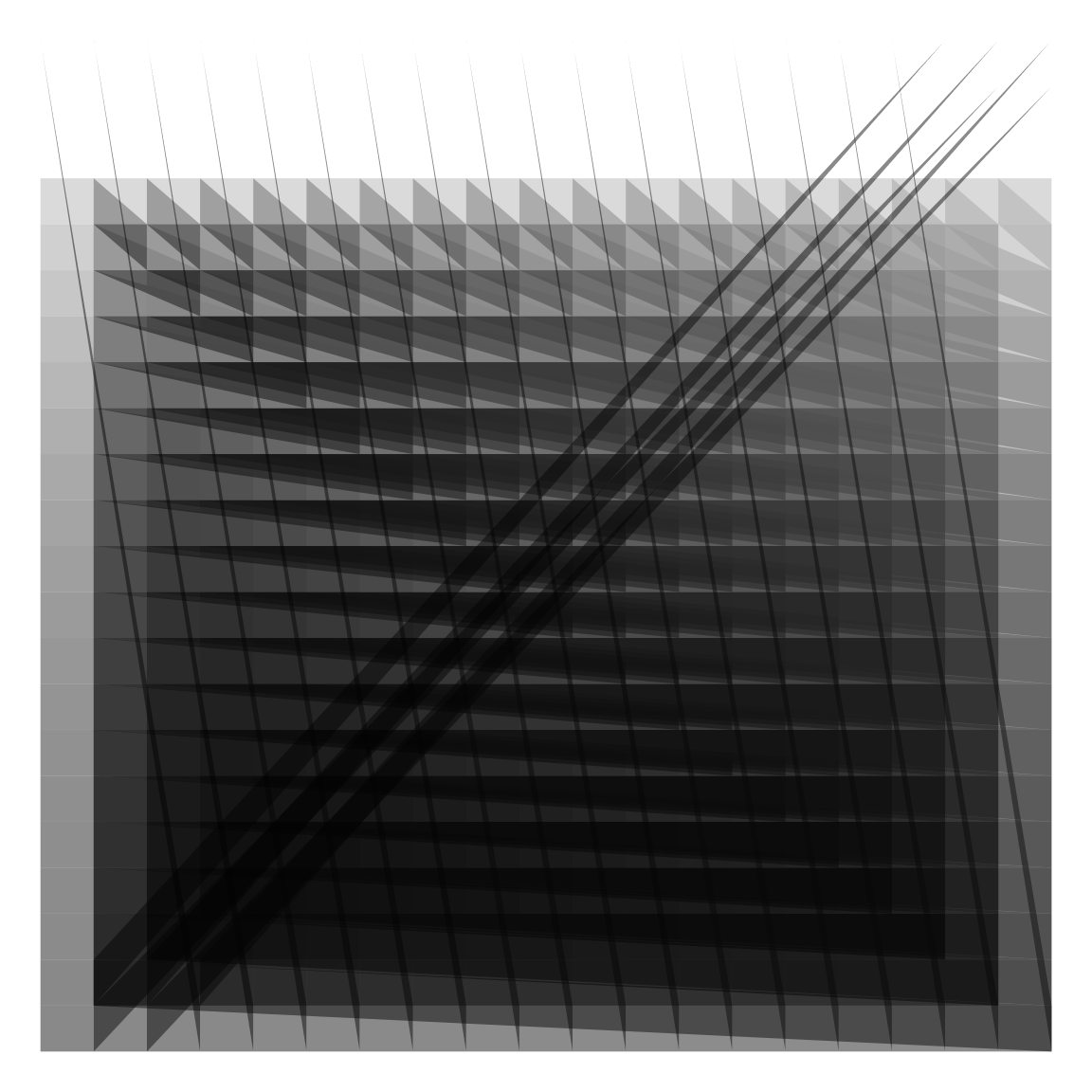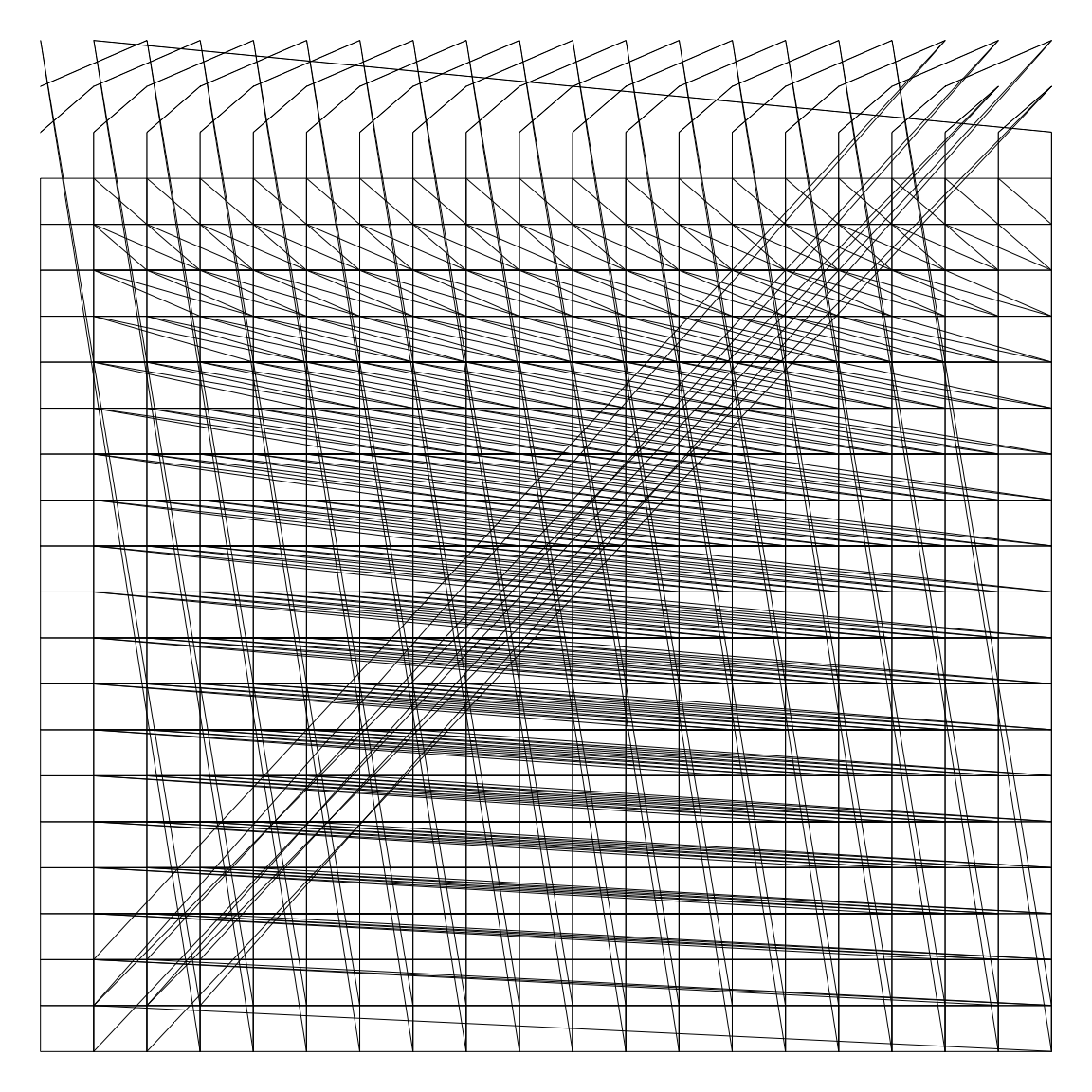Code.

# Shattered Dreams

This one happened late when I thought I was done and it almost broke me. It was a simple fix, but those are hard to find with bleary eyes.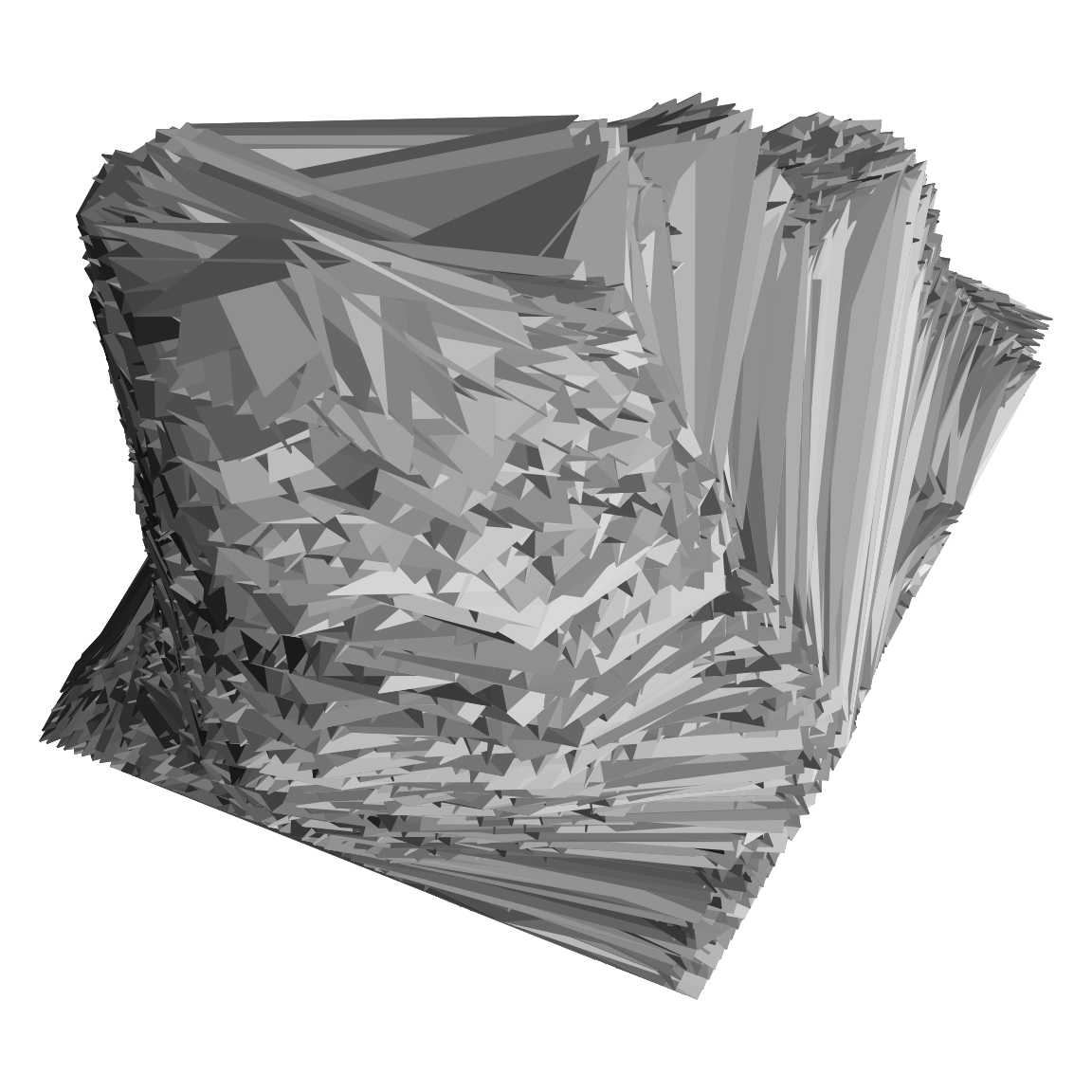Code.

# Stormy Night

You probably wouldn’t guess I was trying my hand at stereoscopy from this one. This was supposed to be a ray-shaded elevation shifted slightly along the y axis for the parallax for stereoscopy. Instead we get storm clouds at night.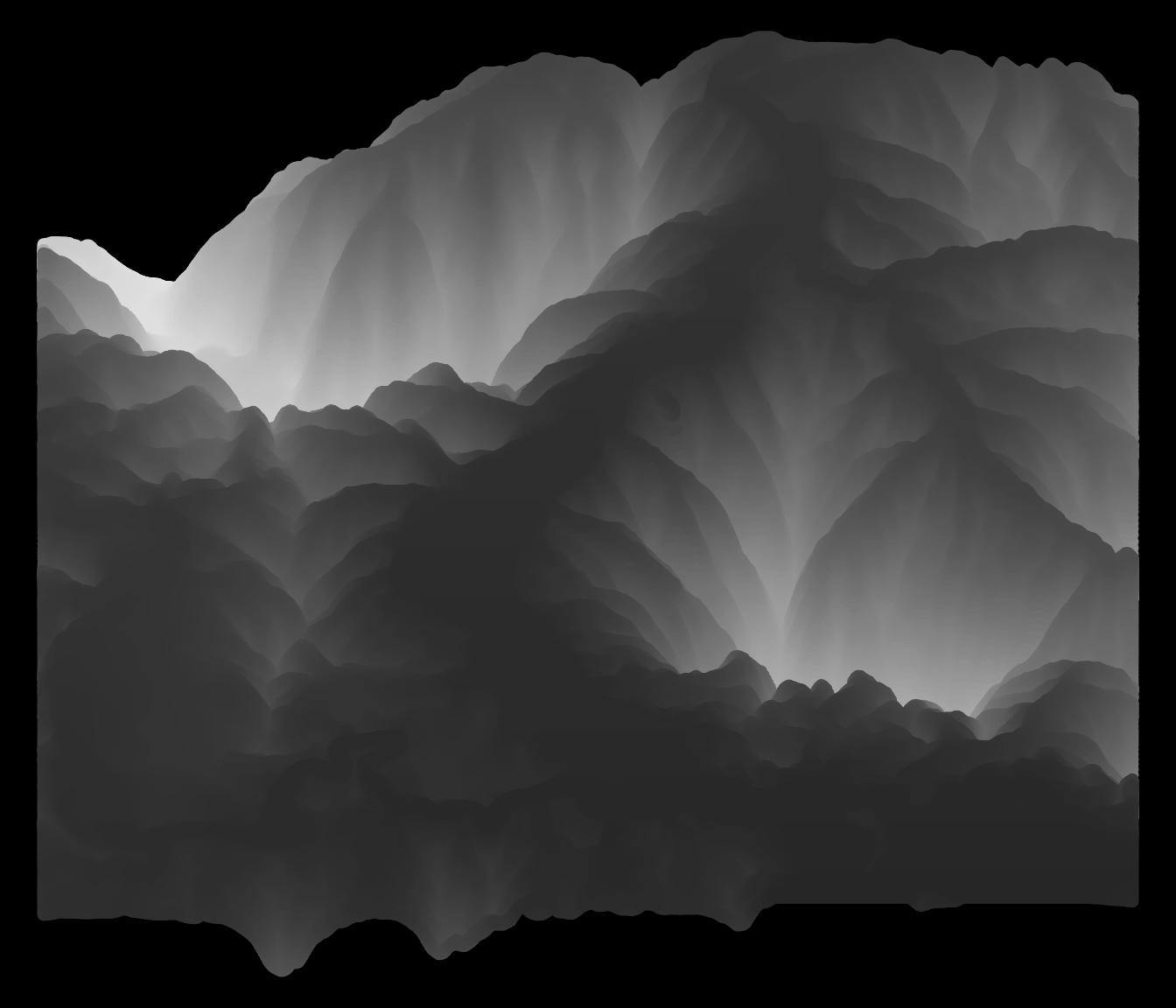Code.

# Static

I find it particularly interesting that some parts of the image are not scrambled.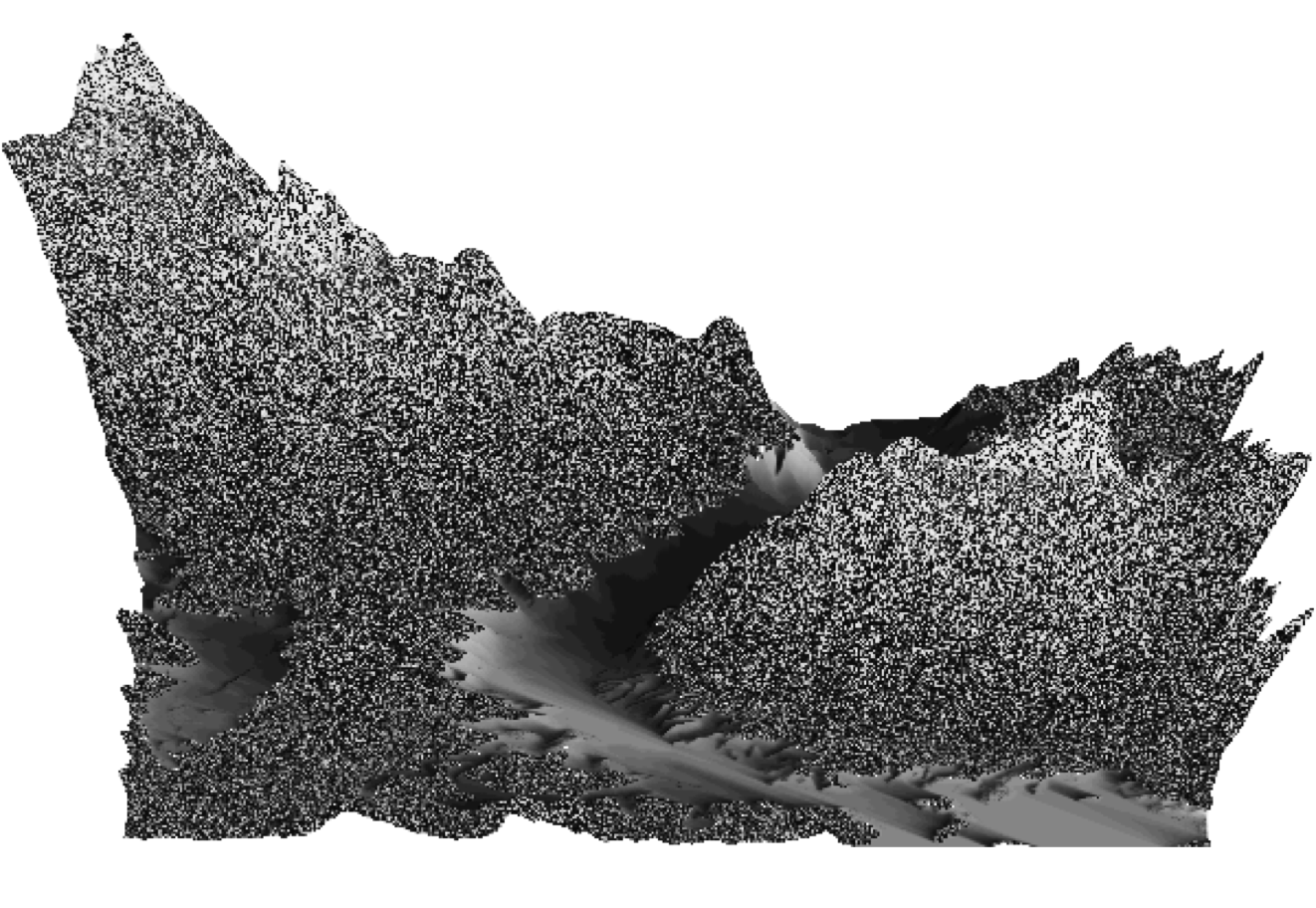The code for this one is lost.

# Orthogonal

A bit banal, but there is something about the glitchy look that I like.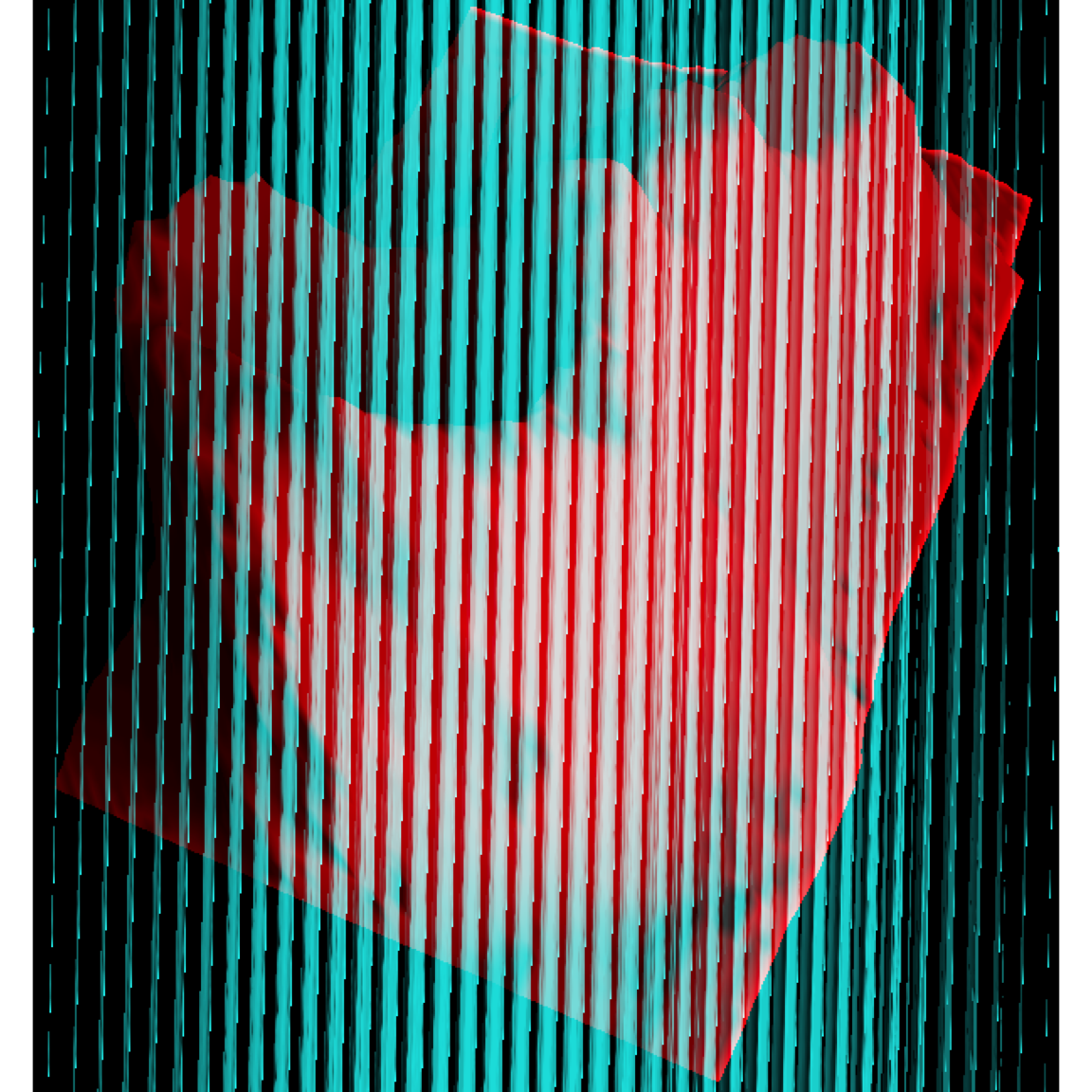Code.

# Conclusions

It’s hard to intentionally reproduce accidental art. If you get something cool be sure to save the code.

# Appendix

## Hash Code

``````nr <- 23
nc <- 20
mx <- matrix(numeric(nr * nc), nc)   # <- BAD, should be ncol=nc
par(mai=numeric(4))
col <- paste0(gray((row(mx[-1,-1]) * col(mx[-1,-1])/((nr-1)*(nr-1)))), "77")
suppressWarnings(
mxpoly.x <- rbind(
c(row(mx)[-nr, -nc]), c(row(mx)[-1, -nc]), c(row(mx)[-1, -1]),
c(row(mx)[-nr, -1]), NA
) )
suppressWarnings(
mxpoly.y <- rbind(
c(col(mx)[-nr, -nc]), c(col(mx)[-1, -nc]), c(col(mx)[-1, -1]),
c(col(mx)[-nr, -1]), NA
) )
plot.new()
polygon(
(mxpoly.x - 1) / (max(mxpoly.x,na.rm=TRUE) - 1),
(mxpoly.y - 1) / (max(mxpoly.y,na.rm=TRUE) - 1),
col=col, border=NA
)
plot.new()
polygon(
(mxpoly.x - 1) / (max(mxpoly.x,na.rm=TRUE) - 1),
(mxpoly.y - 1) / (max(mxpoly.y,na.rm=TRUE) - 1),
border='black', lwd=0.5
)``````

## Shattered Dreams Code

``````library(shadow)
rescale <- function(x, range=1, center=0.5)
((x - min(x, na.rm=TRUE)) / diff(range(x, na.rm=TRUE))) * range +
(1 - range) * center
volc.l <- rbind(x=c(row(volcano)), y=c(col(volcano)), z=c(volcano))
rot <- rot_x(-20) %*% rot_z(65)
volc.lr <- rot %*% volc.l
vl <- lapply(seq_len(nrow(volc.lr)), function(x) volc.lr[x,])
names(vl) <- c('x','y','z')
vlp <- vl
vlp[c('x','y')] <- lapply(vl[c('x','y')], function(x) x - sum(range(x)) / 2)
z.rng <- range(vlp[['z']])
D <- .5
ZD <- diff(z.rng)
vlp[['z']] <- vlp[['z']] - (z.rng + D * ZD)
vlp[['t']] <- ray_shade2(volcano, seq(-90, 90, length=25), sunangle=180)
z.factor <- -1 / vlp[['z']]
vlp[c('x','y')] <- lapply(vlp[c('x','y')], '*', z.factor)
vlp <- sapply(vlp, '[', order(vlp[['z']]), simplify=FALSE)  # <-- BAD
mesh.tri <- mesh_tri(vlp, dim(volcano))
zord <- order(Reduce('+', mesh.tri[,'z']))
x <- do.call(rbind, c(mesh.tri[,'x'], list(NA)))[,zord]
y <- do.call(rbind, c(mesh.tri[,'y'], list(NA)))[,zord]
texture <- gray((Reduce('+', mesh.tri[,'t'])/nrow(mesh.tri)))[zord]
par(mai=numeric(4))
plot.new()
plot.window(c(0,1), c(0,1), asp=diff(range(vlp[['x']]))/diff(range(vlp[['y']])))
polygon(rescale(x), rescale(y), col=texture, border=texture)``````

## Stormy Night Code

``````dem <- readRDS('../../static/data/three-d-pipeline-elev-complex.RDS')
ell <- rbind(x=c(row(dem)), y=c(col(dem)), z=c(dem))
elfin <- rot_y(10) %*% ell
elfin <- elfin[,order(-elfin[3,])]

par(mai=numeric(4), bg='black')
plot.new()
points(
y=rescale(-elfin[1,]), x=rescale(elfin[2,]),
col=gray(rescale(elfin[3,], .8, 1)),
pch=16
)``````

## Orthogonal Code

``````rot <- rot_x(-20) %*% rot_z(65)
rot.l <- rot %*% rot_z(2.5)
rot.r <- rot %*% rot_z(-2.5)